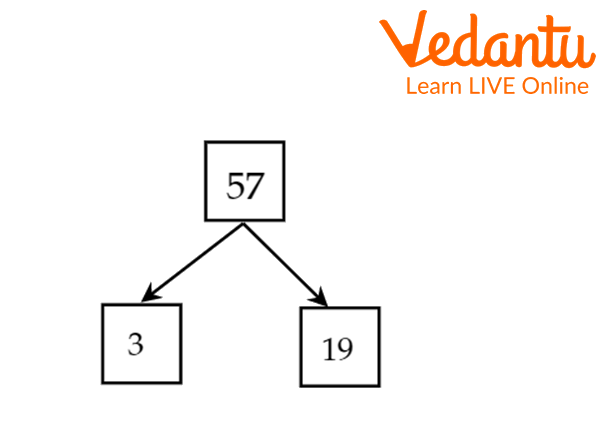Courses
Courses for Kids
Free study material
Free LIVE classes
More

# Factor of 57## Factor of 57: An Introduction

If 3 people have to share Rs 57 equally, then when we divide 57 by 3, we get 19, and every person gets Rs 19. Therefore, 57 is divisible by 3 and 19, or we can say 3 and 19 are the factors of 57. In other words, dividing any given number by such a number that divides it completely (the remainder is 0) is called the factor of that number. Here, factors of 57 are 5, 19, 3 and 1.

## Factors of 57

The factors of a number exactly divide the given number. The factors of 57 are the numbers that divide 57 completely and leave the remainder 0. In other words, we can say that the factors of 57 are those numbers when multiplied in pairs, giving the the original number, i.e. 57. If a number can be expressed as a product of two numbers, then the numbers are a factor of that number.

Here the number is 57, which is a multiplication of 3 and 19, which can be written as $3\times 19=57$, Here, 3 and 19 divide 57 without leaving any remainder. Hence 1,3,19 and 57 are all factors of 57; similarly, -1,-3,-19 and -57 are the negative factors of 57.

## Prime Factorization of 57

First, we will understand what a prime number is. A prime number is greater than 1 that can only be divided by 1 or itself. So, a prime number has only two factors,1 and the number itself. For example, 2, 3, 5 and 7 are prime numbers.

Now come to the prime factorisation of 57. By prime factorization of 57, we will know what the factors of 57 are. As we know, prime factorisation is writing a composite number as a product of prime numbers. There are two methods of prime factorisation 57:

1. Division Method

2. Factor Tree Method

## Division Method to Find Prime Factors of 57

In this method, we start dividing by the smallest prime number. We keep on dividing by prime numbers till we obtain 1 as the quotient.

57 is an odd number, so it can not be divided by 2. We divide 57 by 3

Step 1—> $57\div3=19$. The quotient is 19, Remainder is 0. 19 is a prime number, so it is divisible by 19 and 1.

Step 2—> $19\div 19=1$. We finally get the quotient 1. The remainder is 0

 3 57 19 19 1

Hence the Prime Factorization of $57=3\times19$

## Factor Tree Method to Find Factors of 57

In the Factor tree method, we factorise the given composite number till we get all the prime factors. Prime factorisation of $57=3\times19$.Factor tree of 57

Hence the prime factorisation of $57=3\times 19$.

## Pair of Factors

The pair of factors 57 are those numbers when multiplied together, give 57. A number has a negative and positive pair of factors.

The pair of factors of 57 are as follows:

 Positive Factors of 57 Positive Pair of factors of 57 $1\times57$ (1,57) $3\times19$ (3,19)

 Negative Factors of 57 Negative Pair of factors of 57 $-1\times(-57)$ (-1,-57) $-3\times(-19)$ (-3,-19)

Hence, the positive pair of factors are (1,57) & (3,19) and the negative pair of factors are (-1,-57) & (-3,-19).

## Solved Examples

Q1 Find the common factors of 57 and 19.

Solution: We do this question by prime factorization method

$19=1\times 19$

$57=1\times 3 \times 19$

The common factor of 19 and 57 is 19.

Q2 Find the prime common factors of 171 and 228 .

Solution:

 3 171 3 57 19 19 1

The prime factors of 171 are 3 and 19.

 2 228 2 114 3 57 19 19 1

The prime factors of 228 are 2,3, and 19.

Hence the common prime factors of 171 & 228 are 3 and 19.

Q3 Factorise the 48 by division method.

Solution:

Step 1: Start dividing 48 by 2, the smallest prime number.

Step 2: $48\div 2=24$. The quotient is 24, which is divisible by 2.

Step 3: $24\div2=12$. The quotient is 12, which is divisible by 2.

Step 4: $12\div2=6$. The quotient is 6, which is again divisible by 2.

Step 5: $6\div 2=3$. We now get the quotient 3, a prime number itself. It is divisible by 3 only.

Step 6: $3\div 3=1$, We finally get the quotient 1.

 2 48 2 24 2 12 2 6 3 3 1

Hence the Prime Factorization of $48 = 2 \times 2 \times 2 \times 2 \times 3$

## Key Features

• The factors of a number exactly divide the given number.

• The factors of 57 are the numbers that divide 57 completely and leave the remainder 0.

## Practice Problems

Q1. What is the number of positive factors of 13?

Q2. What are the prime factors of 34?

## List of Related Articles

Last updated date: 26th May 2023
Total views: 2.1k
Views today: 0.01k

## FAQs on Factor of 57

1 Does a factor of a number completely divide the number?

A factor of a number is a number that divides the number without leaving a remainder. Thus, a factor of a number completely divides the number.

2 How many prime factors do 57 have?

57 has 2 prime factors: 3 and 19.

3 Are the multiples and the factors of a number the same?

A factor of a number must be less than or equal to the number. A multiple of a number must be greater than or equal to the number. The number itself is a  factor and a multiple of the number.